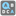• # func.sort() & sorted(func)

## Question related to missionCommon Words

def checkio(first, second): first = first.split(",") second = second.split(",") same = [i for i in first if i in second] return ",".join(i for i in sorted(same)) if same else ""

This is how I passed the project but my question is why this code is wrong?

def checkio(first, second): first = first.split(",") second = second.split(",") same = [i for i in first if i in second].sort() return ",".join(i for i in same) if same else ""